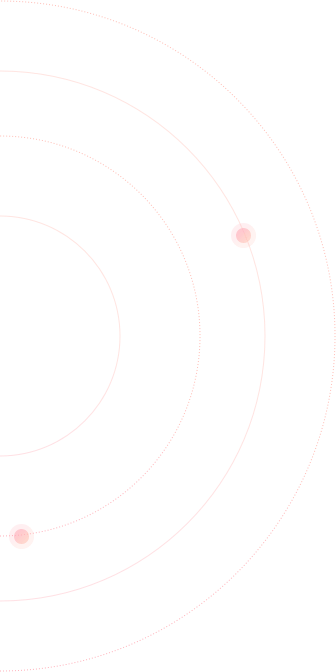created : 2 years ago| |  live deployment: 0

Fibonacci Pivot Points Template

There are no tags defined for this strategy.

Strategy description

Fibonacci Pivot Points

P = (High + Low + Close)/3

S1 = P – (0.382 * (High – Low))

S2 = P – (0.6182 * (High – Low))

S3 = P – (1 * (High – Low))

R1 = P + (0.382 * (High – Low))

R2 = P + (0.6182 * (High – Low))

R3 = P + (1 * (High – Low))

Subscribers

View all